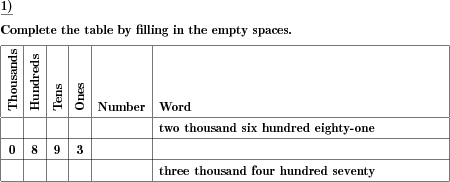Custom math worksheets at your fingertips# Details for problem "Place value board table and number words"

Quickname: 5653

Elementary School, Primary School, Middle School.

## Summary

In a table, place values and number words have to be added

## Example## Description

Place value is a key concept to understand numbers and the whole Maths rely on this topic, this skill can be mastered using these worksheets. A table is presented with the place values of thousands, hundreds, tens, and ones, plus columns for the number and number word. The place values or the number word are given, and the other values have to be filled in.

A wonderful idea to practice and develop an understanding of place values are included in this worksheet. These worksheets can be used in whole-class activity to discuss and clear concepts of learners from grade 3 to grade 5. These worksheets aim to develop the following set of skills in learners.

1. Identify the value of a digit by observing its place.
2. Able to understand the place value of a number and place it in the table accordingly.
3. Understand the mathematical concepts more deeply and widely.
4. Convert the digits on places to a number in words.

• The number of lines to be solved is selectable.
• The range of numbers can be chosen from 99 to 9999.
• Depending on the number range chosen, only the columns hundreds, tens, and ones, or only the columns tens and ones will be shown.

The answer key provides all missing places filled.

Download free printable worksheets for this math problem here. The worksheet contains the problems only, the solution sheet includes the answers. Just click on the respective link.

•Worksheet 1Solution sheet with answers
•Worksheet 2Solution sheet with answers
•Worksheet 3Solution sheet with answers

If you can not see the solution sheets for download, they may be filtered out by an ad blocker that you may have installed. If this is the case, please allow ads for this page and reload the page. The solution sheets will then reappear.

• Do these sample worksheets do not really fit?
• Do you need more math worksheets, with a different level of difficulty?
• Would you like to combine different problems on a worksheet and adjust them to your needs?
• As a teacher, you can put together your own worksheets using the automatically generated math problems provided.
With a free initial credit, you can start creating your own math worksheets in a few minutes.

You can try it for free! Register here, to create custom worksheets now!

## Customization options for this problem

Parameter
Possible values
Number of rows
1, 2, 3, 4, 5, 6, 7, 8, 9, 10
Range
99, 999, 9999
Columns given
Mixed, Place values, Number wordDeutsche Version dieser Aufgabe
These informational pages with samples describe math problems that can be combined on custom math worksheets with solutions for home and K-12 school use.
Deutsche Seiten
×